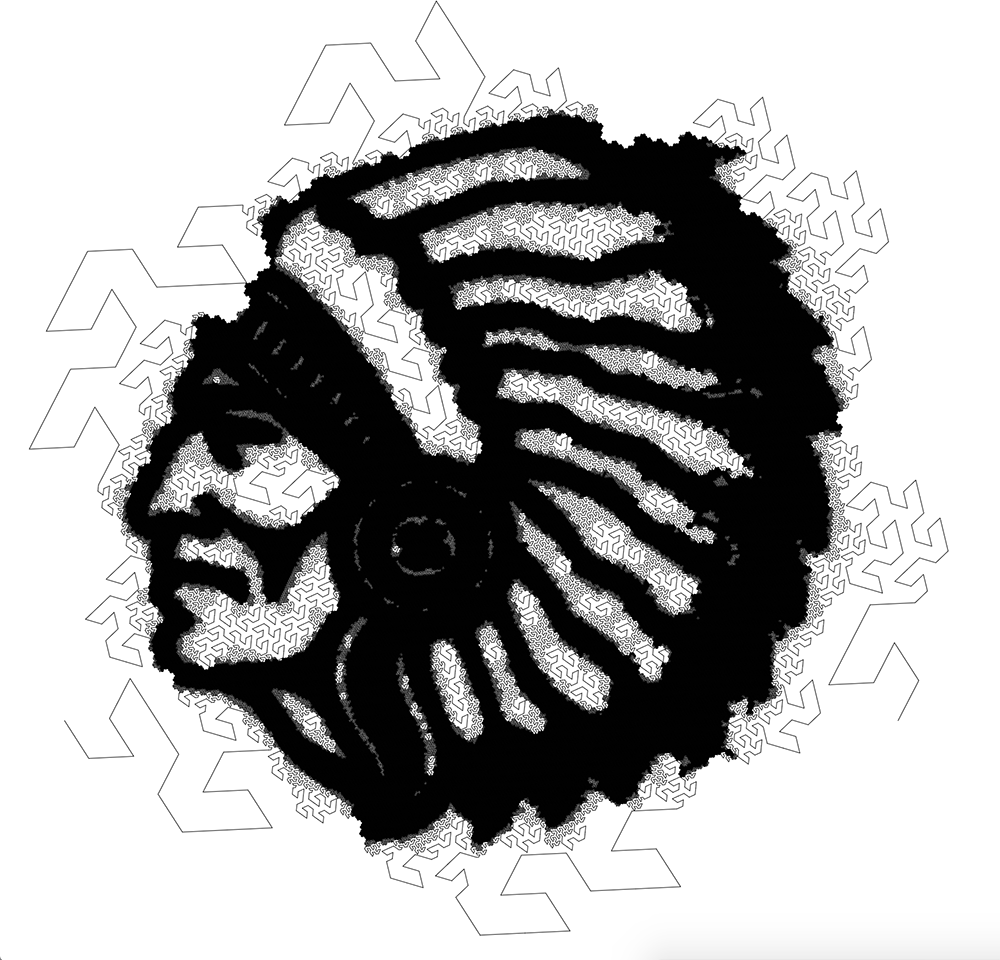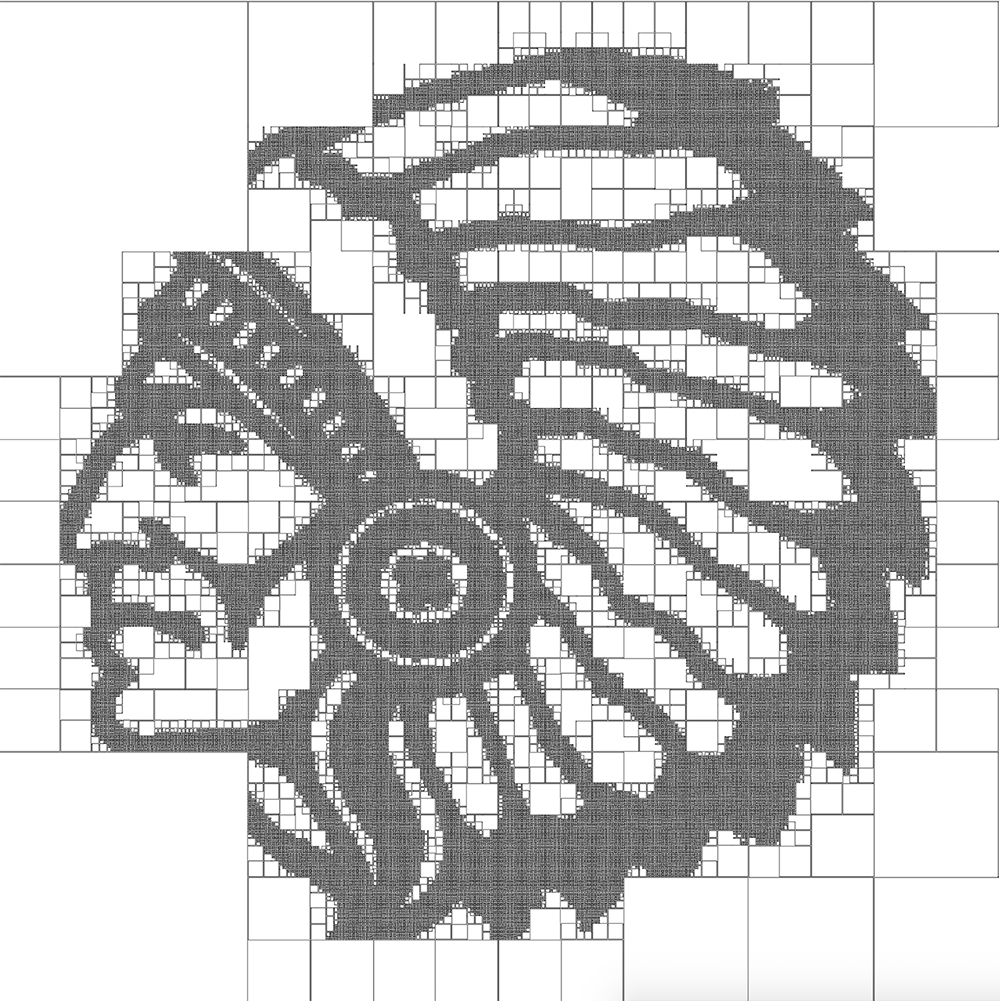# Space Filling CurvesSpace Filling Curves is an “image based space-filling curve generator”.

It’s a sort of artwork.

Drag’n’drop the image of your choice on the canvas to generate a hilbert or gosper curve version.

## How does it work?

A Space Filling Curve (Hilbert curve or a Gosper curve) is computed from a grayscale image, refined where the image is darker than thredhold.

### General idea

The image is recusively divided in tiles.

• Generate image mipmaps: pre-calculated, optimized sequences of images, each of which is a progressively lower resolution representation of the same image
• Subdivide the image with the lowest resolution in tiles and for each tile:
• if the tile is darker than threshold:
• resubdivide in tiles and continue recursively until the tile is light enough
• otherwise draw the corresponding curve at proper scale

### Hilbert

The implementation is much more specific than the Gosper curve one, thus less elegant and more complicated.

For the Hilbert curve, the “tiles” correspond to four quadrant, which means the image is recusively divided in four quadrants, numbered from 0 to 3.

The goal is to compute the traversing order of the quadrants (for example [0, 1, 2, 3], [3, 0, 1, 2], etc.).

The tricky part is to rotate the curve in the proper way when it must be refined.

The first and last quadrant must be rotated in opposite direction, the traversing order of the quadrants must be inverted, and depending on the previous refinements the rotations must happen in one way or another.

The final algorithm keeps track of the rotation which sums up at each iteration, and whether or not rotation inversion must happen.

At each iteration, the rotation and inversion is computed in the following way: